Part4 函数定义

1.PUTS

TABLE_HINT_STRING

TABLE_HINT_STRING

6.PUTS

TABLE_HINT_STRING

运行截图

输入学生信息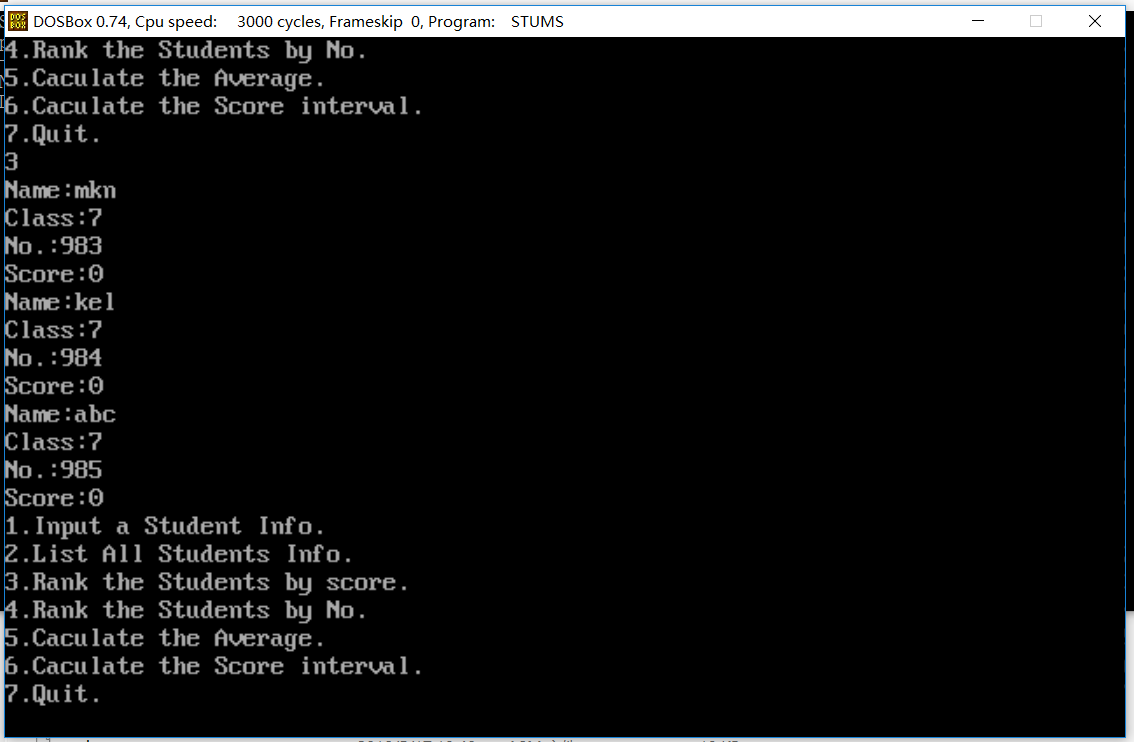列出所有学生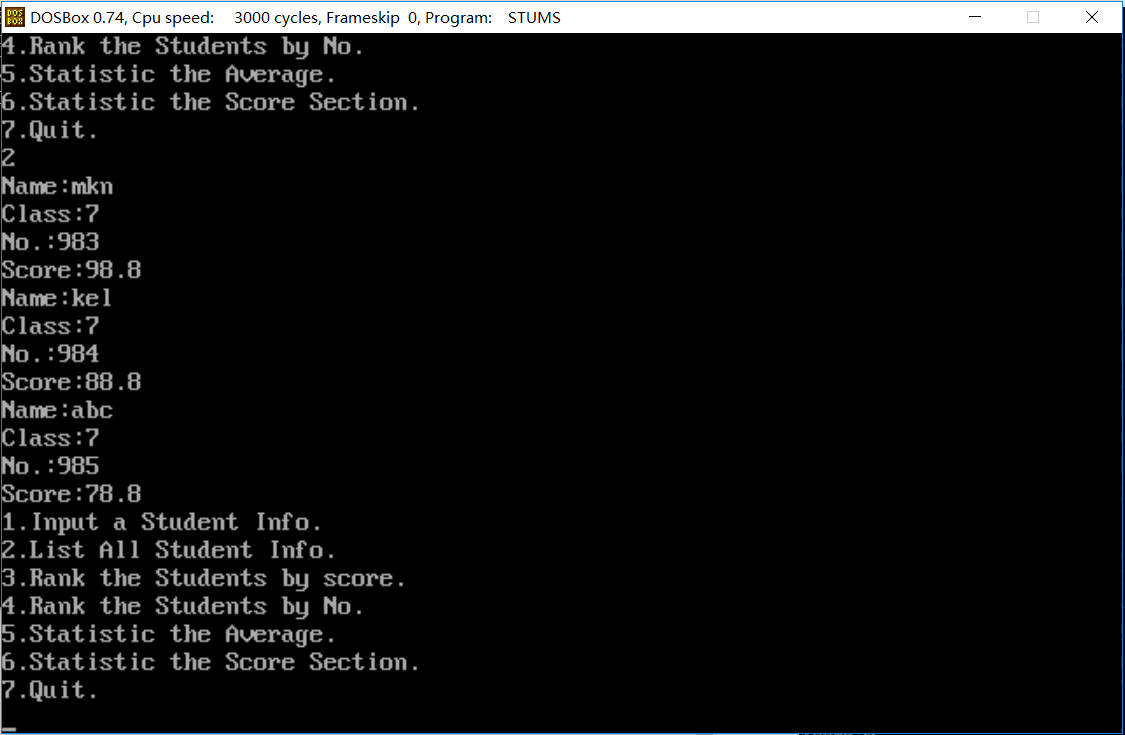按成绩排名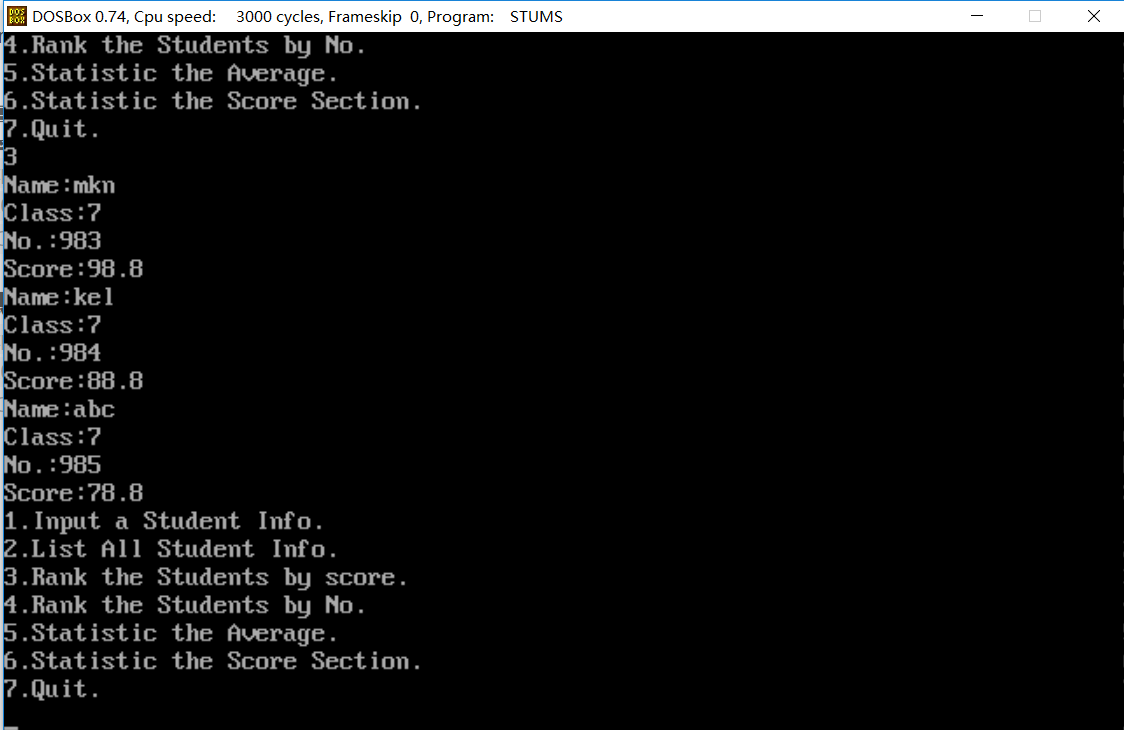按学号排名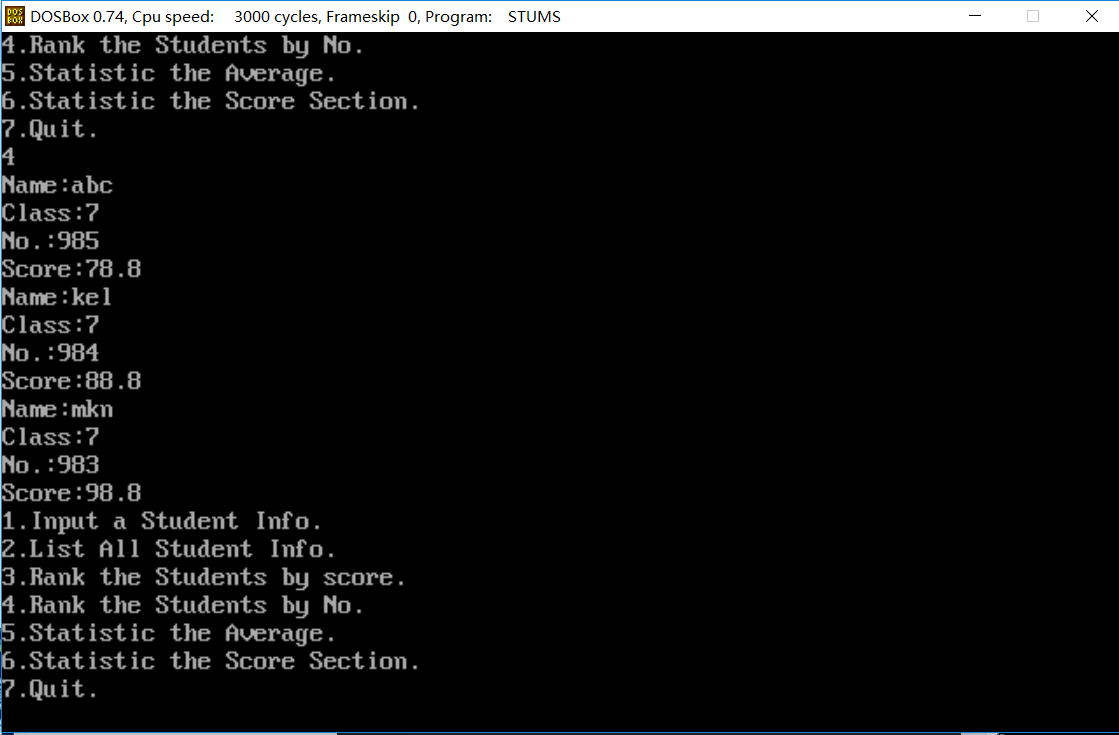计算平均分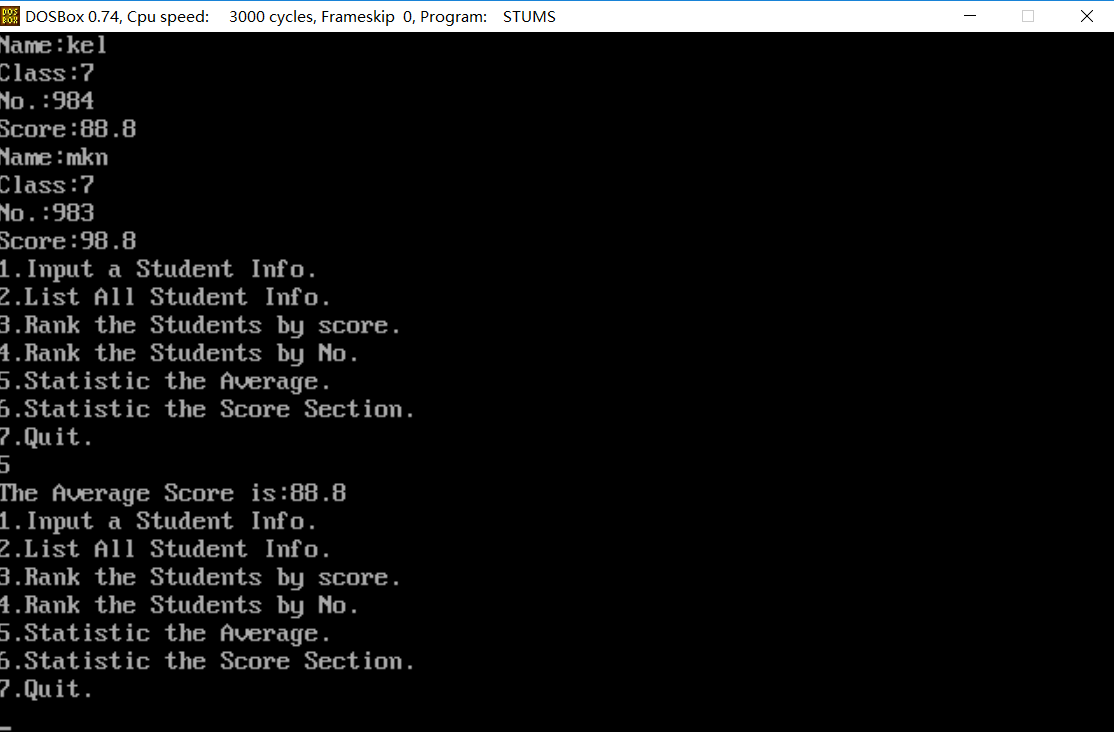划分成绩区间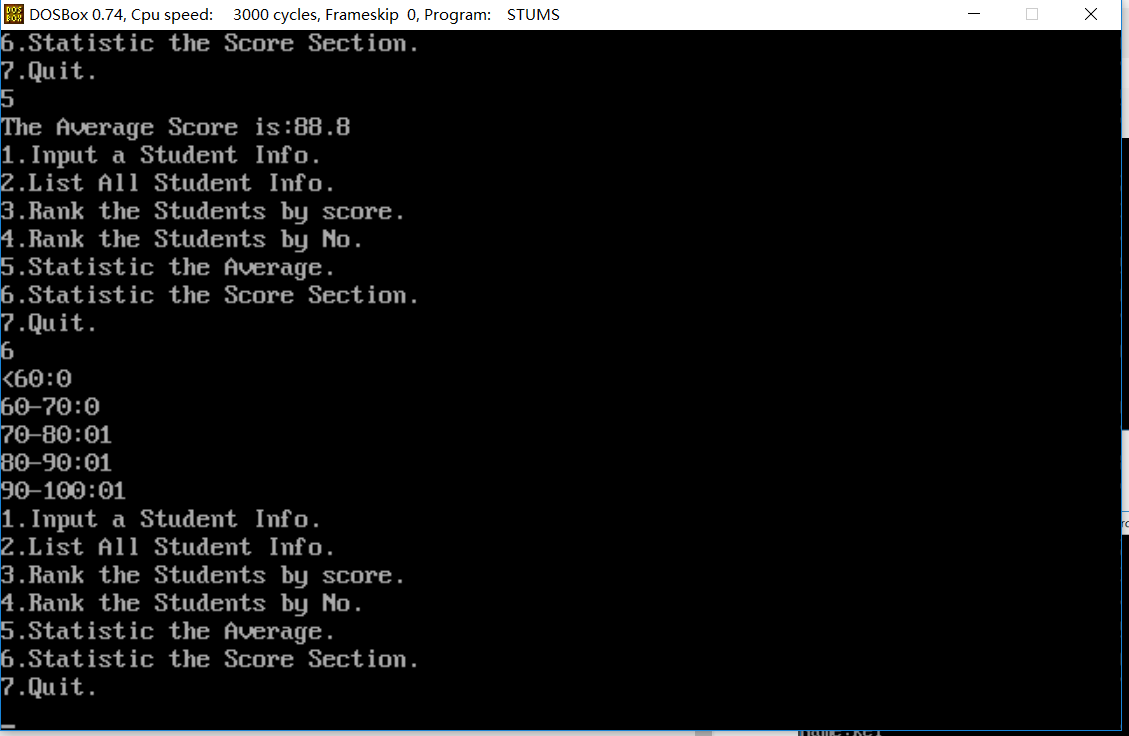编写过程中的问题

1.跳转表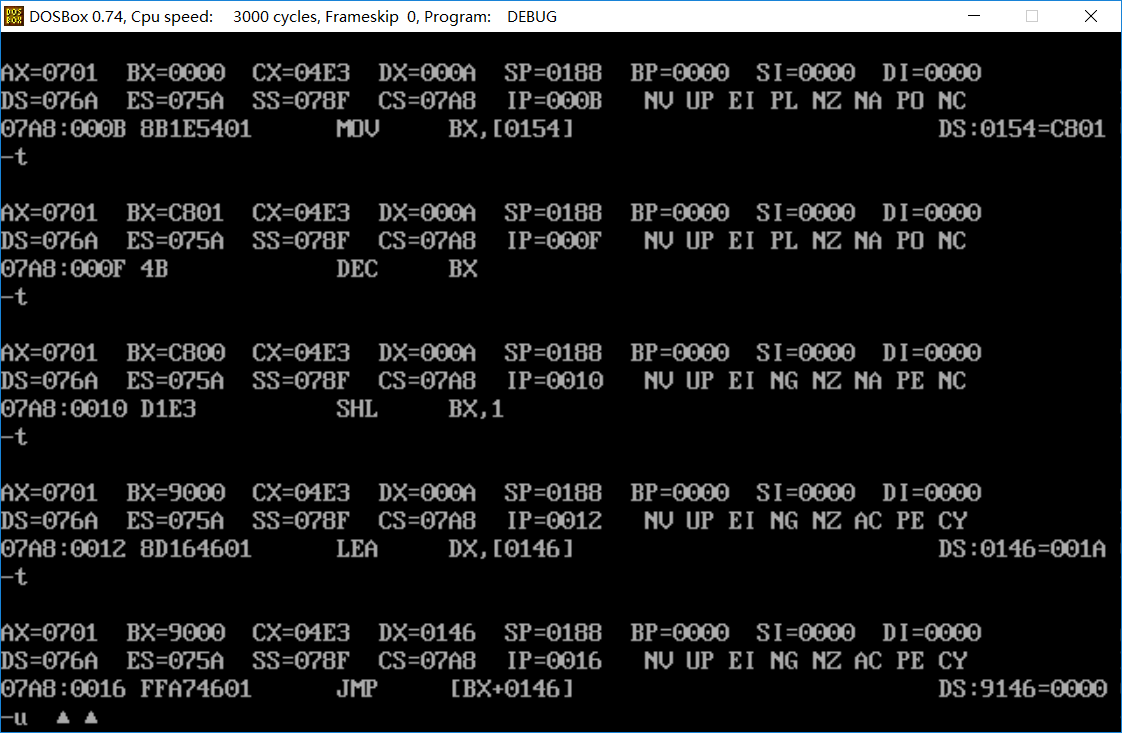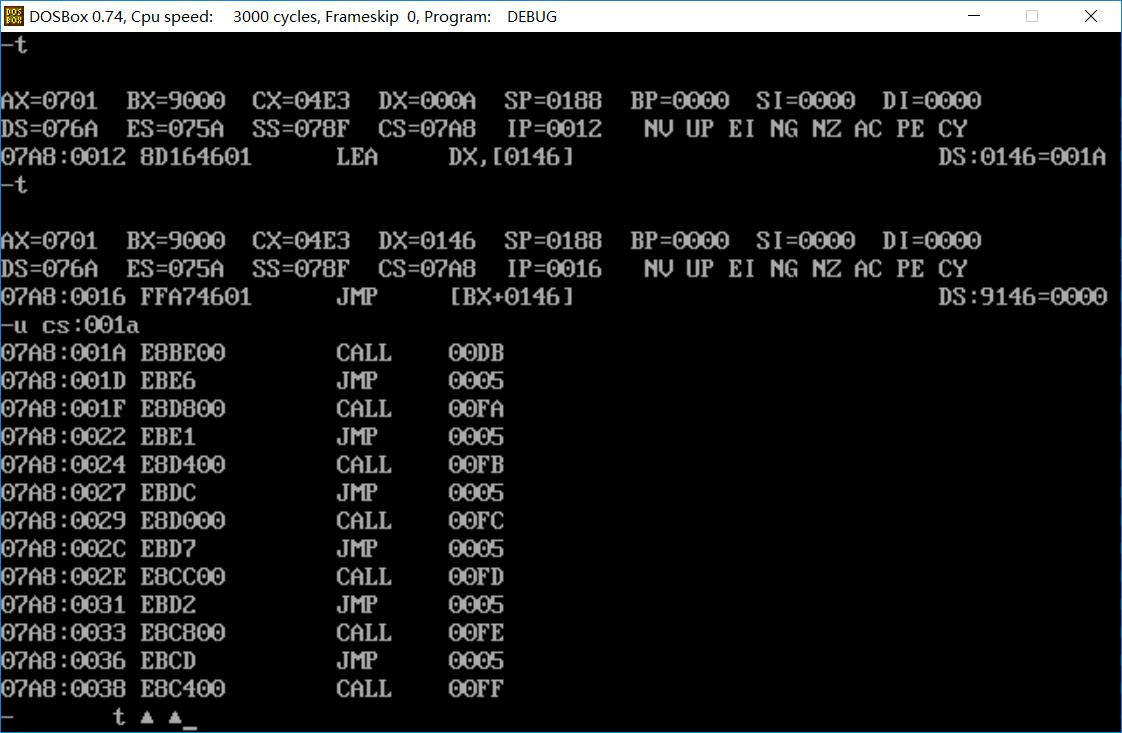为什么会有这样一个工具？

<p>
之前是有一位学长写了JS版和Python版的评教脚本，不过自从教务系统加了验证码后就废弃无人维护了。

众所周知，北邮的教学评估总是安排在期中期末复习周，然而，凡是使用过教学评估界面的同学都能理解在评教界面上找到选项并点击有多困难。

<br>更可怕的是,每个老师通常都会有7~8个选项，一个一个点下来眼睛都要瞎了。<br>
鉴于此，为了解决这个问题，我写了这样一个“糙猛快”的脚本，来帮助大家一键评教。
</p>

写在最后

1.因为是突发奇想写的，所以可能会存在一些问题，使用时遇到问题记得先问问谷歌

2.还是由于突发奇想写的，界面十分简陋，其实验证码可以通过OCR解决的，不过懒得折腾，有想法的同学自己折腾一下吧。最好能部署成云服务的形式，使用更方便，用户也就不用配置各种依赖了。

72.Edit distance

Description

Given two words word1 and word2, find the minimum number of steps required to convert word1 to word2. (each operation is counted as 1 step.)

You have the following 3 operations permitted on a word:

a) Insert a character

b) Delete a character

c) Replace a character

class Solution {
public int minDistance(String word1, String word2) {
int [][]dp=new int[word1.length()+1][word2.length()+1];
for(int i=0;i<=word1.length();++i){
for(int j=0;j<= word2.length();++j){
if(i==0||j==0){
dp[i][j]=i+j;
}
else{
if(word1.charAt(i-1)==word2.charAt(j-1))
dp[i][j]=dp[i-1][j-1];
else{
int replace=dp[i-1][j-1];
int delete=dp[i-1][j];
int insert=dp[i][j-1];
dp[i][j]=Math.min(Math.min(replace,delete),insert);
dp[i][j]++;
}
}
}
}
return dp[word1.length()][word2.length()];
}
}

583.Delete Operation for Two Strings

Description

Given two words word1 and word2, find the minimum number of steps required to make word1 and word2 the same, where in each step you can delete one character in either string.

Example 1:

Input: "sea", "eat"
Output: 2
Explanation: You need one step to make "sea" to "ea" and another step to make "eat" to "ea".

Note:

The length of given words won’t exceed 500.

Characters in given words can only be lower-case letters.

cnblog和belong，最长公共子序列是blog，而最长公共子串是lo

public class Solution {
public int minDistance(String s1, String s2) {
return s1.length() + s2.length() - 2 * lcs(s1, s2, s1.length(), s2.length());
}
public int lcs(String s1, String s2, int m, int n) {
if (m == 0 || n == 0)
return 0;
if (s1.charAt(m - 1) == s2.charAt(n - 1))
return 1 + lcs(s1, s2, m - 1, n - 1);
else
return Math.max(lcs(s1, s2, m, n - 1), lcs(s1, s2, m - 1, n));
}
}

class Solution {
public int minDistance(String word1, String word2) {
int dp[][]=new int[word1.length()+1][word2.length()+1];
for(int i=0;i<word1.length()+1;++i){
for(int j=0;j<word2.length()+1;++j){
if(i==0||j==0)
continue;
if(word1.charAt(i-1)==word2.charAt(j-1))
dp[i][j]=dp[i-1][j-1]+1;
else
dp[i][j]=Math.max(dp[i-1][j],dp[i][j-1]);
}
}
return word1.length()+word2.length()-2*dp[word1.length()][word2.length()];
}
}

class Solution {
public int minDistance(String word1, String word2){
int dp[][]=new int[word1.length()+1][word2.length()+1];
for(int i=0;i<=word1.length();++i){
for(int j=0;j<=word2.length();++j){
if(i==0||j==0)
dp[i][j]=i+j;
else if(word1.charAt(i-1)==word2.charAt(j-1))
dp[i][j]=dp[i-1][j-1];
else
dp[i][j]=Math.min(dp[i-1][j],dp[i][j-1])+1;
}
}
return dp[word1.length()][word2.length()];
}
}

521. Longest Uncommon Subsequence I

Description

Given a group of two strings, you need to find the longest uncommon subsequence of this group of two strings. The longest uncommon subsequence is defined as the longest subsequence of one of these strings and this subsequence should not be any subsequence of the other strings.

A subsequence is a sequence that can be derived from one sequence by deleting some characters without changing the order of the remaining elements. Trivially, any string is a subsequence of itself and an empty string is a subsequence of any string.

The input will be two strings, and the output needs to be the length of the longest uncommon subsequence. If the longest uncommon subsequence doesn’t exist, return -1.

Example 1:

Input: "aba", "cdc"
Output: 3
Explanation: The longest uncommon subsequence is "aba" (or "cdc"),
because "aba" is a subsequence of "aba",
but not a subsequence of any other strings in the group of two strings.

Note:

1.Both strings’ lengths will not exceed 100.

2.Only letters from a ~ z will appear in input strings.

1.“aba”,”cdc”,由于两者之间互不为子串，所以返回两者之中的最长字符串的长度即可

2.“aba”,”babac”,由于aba是后者的子串，但后者却不是前者的子串，所以返回较长的即可

3.两者中有一个为空串，由于题目说明空串是任何字符串的子串，所以依然返回较长的即可

4.两字符串相等，如“aaa”,”aaa”,找不到某一个子串同时不为另一个字符串的子串，所以返回-1

public class Solution {
public int findLUSlength(String a, String b) {
if(!b.contains(a)&&!a.contains(b))
return Math.max(a.length(), b.length());
if(a.contains(b)&&b.contains(a))
return -1;
if(a.contains(b)||b.contains(a))
return Math.max(a.length(), b.length());
if(("".equals(a)&&!"".equals(b))||(!"".equals(a)&&"".equals(b)))
return Math.max(a.length(), b.length());
else
return 0;
}
}

one-line版本的如下：

public class Solution {
public int findLUSlength(String a, String b) {
return a.equals(b)? -1 : Math.max(a.length(),b.length());
}
}